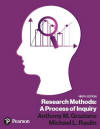﻿ Linear Regression in SPSS for WindowsGraziano & Raulin
Research Methods (9th edition)

## Linear Regression

Linear regression computes the equation for the best fitting straight line for the data. In the scatter plot prepared for the relationship between age and income, you can see that the points do seem to cluster around an imaginary line from the lower left to upper right part of the graph. Regression will compute the optimal values for such a straight line, in which the equation for the line is:

Y' = bX + c

Y' is the predicted score; b is the slope of the best fitting line, and c is the constant for the best fitting line.

To run a regression analysis, select the Analyze menu, the Regression submenu, and the Linear option, which will give you this screen. To compute the best fitting equation for the line for predicting income based on age, move the variable age to the independent variable box and the variable income to the dependent variable box and click on OK, which will give you this output

At the very bottom of this output are the values for "b" and "c" in the above equation (613 and 19,848, respectively) both rounded to whole numbers. That means the income can be predicted with the following equation:

Income = (613 * Age) + 19,848

We would therefore predict that a 20-year-old person would make \$21,108 and a 50-year-old person would make \$50,498. If you inspect the scatter plot for these data, you will see that these predictions are quite close to the actual data for people of those ages.

### Animations

We have prepared an animation that will walk you through this procedure. To run the animation, simply click on the title of the animation in the table below.

Note that we do not recommend that you try to run the animations if you have a slow connection, such as a dial-up connection. You will find that the animations take forever to load with a slow connection.